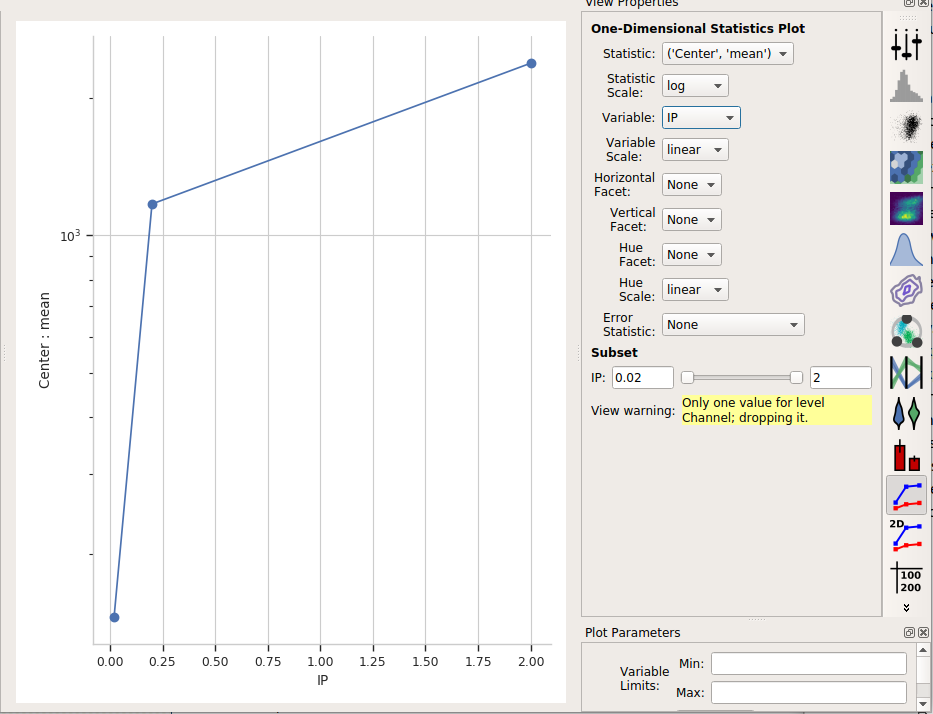# HOWTO: Use different gates for different subsets of data¶

Sometimes, it makes sense to apply different gates to differen subsets of your data set. It’s particularly useful when we’re estimating the gate parameters from the data. For example, consider the following data (taken from the Yeast Dosage-Response Curve tutorial.)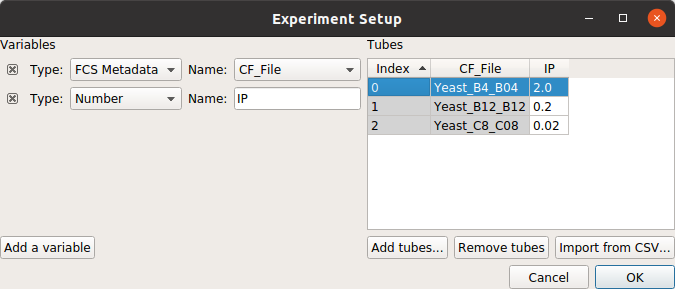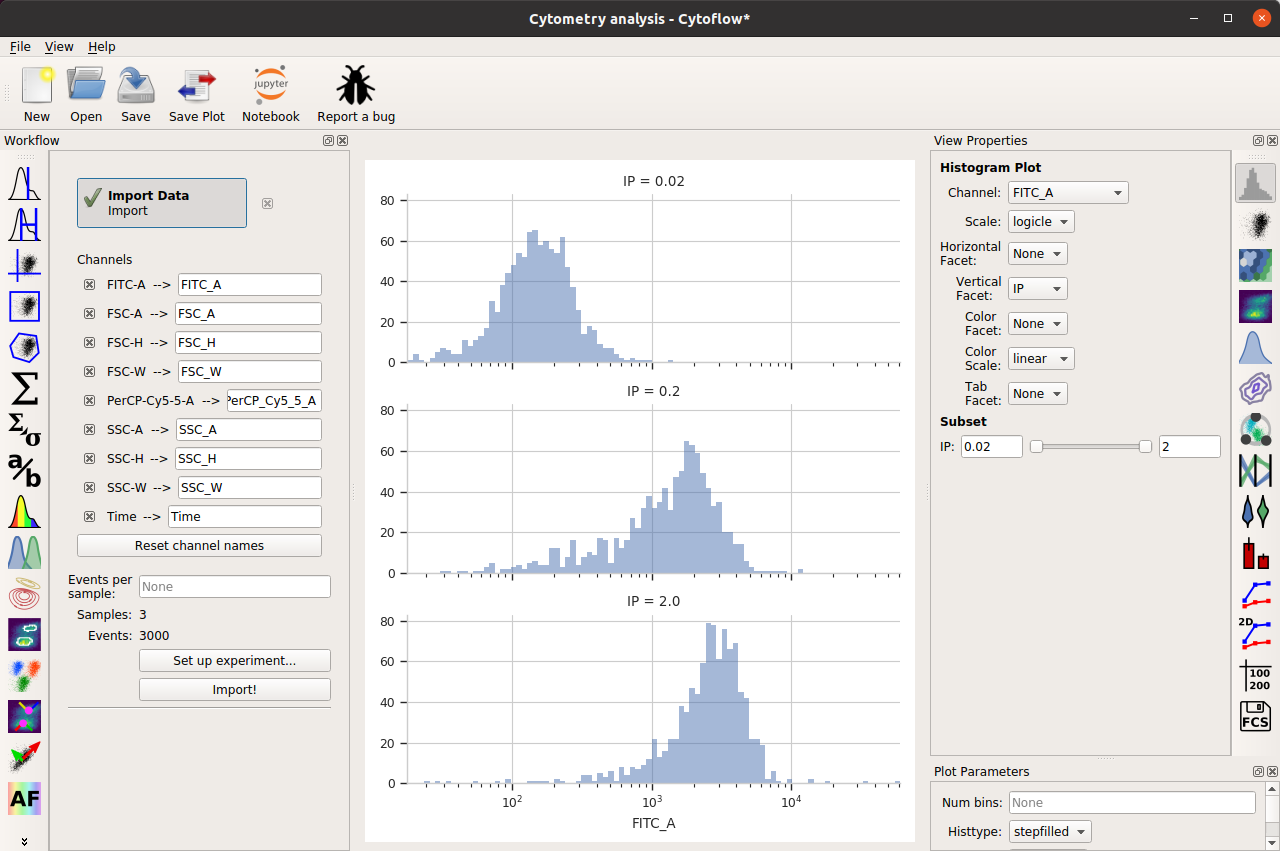Imagine that we want to use the 1D Gaussian operation to choose the events from the center +/- 0.5 standard deviation this data. It’s clear that a gaussian model that fits the first data set won’t fit the second or third!

To address this problem, most data-driven gates have a Group estimates by option. Set this to a condition, and the operation will subdivide the data by that condition and estimate separate model parameters for each subset. For example, if we choose IP as the group-by condition: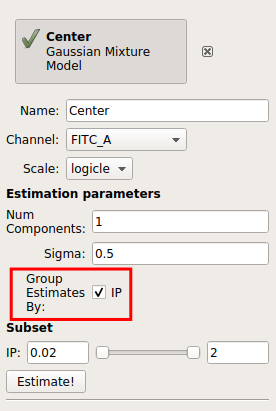Then the operation will give us three different estimates, one for each value of IP: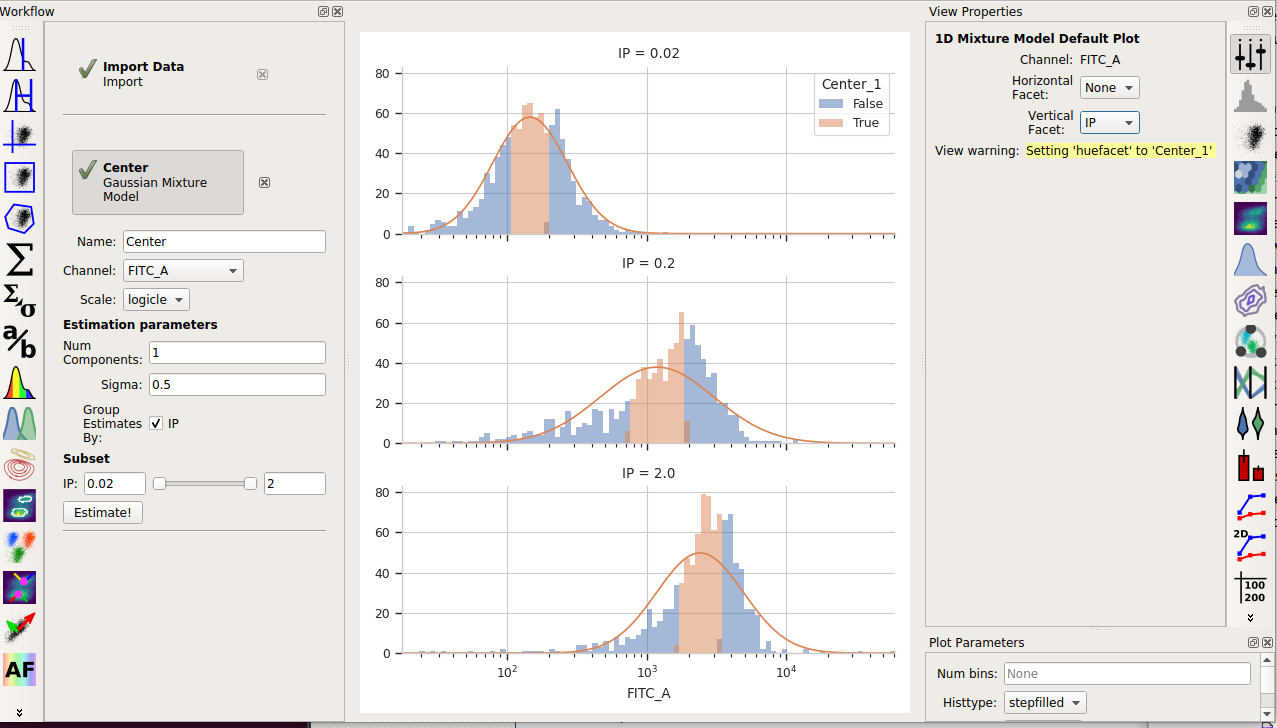And of course, don’t forget that the operation also makes a new statistic containing the means: